Question

Castigliano’s Theorem

```Determine the horizontal and vertical displacement at point E (in millimeters). E = 200 GPa. All
the bars have an area of 5000 mm2```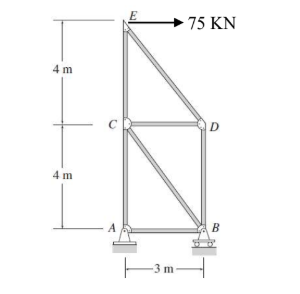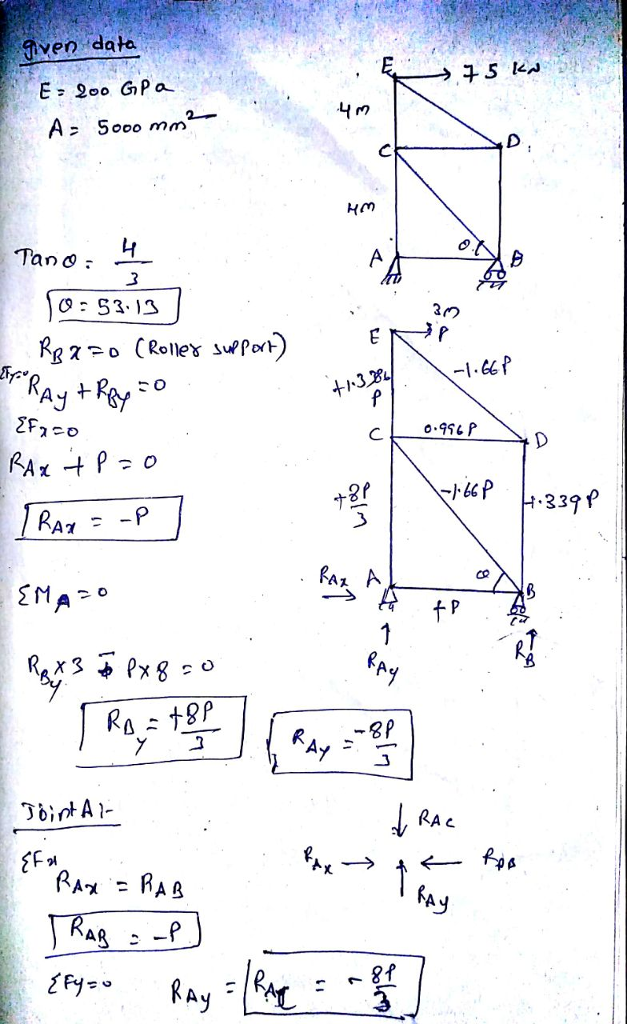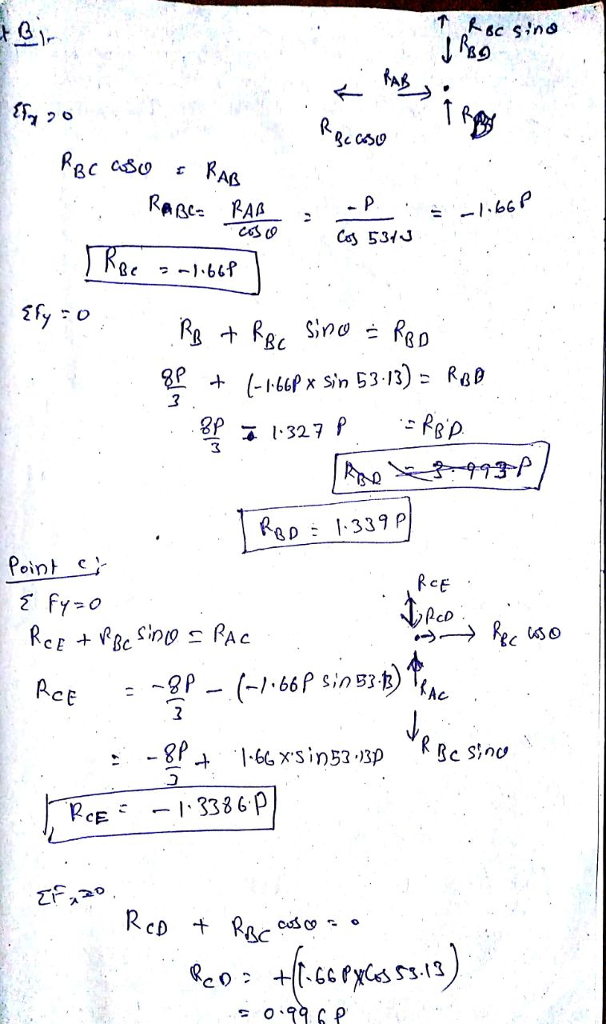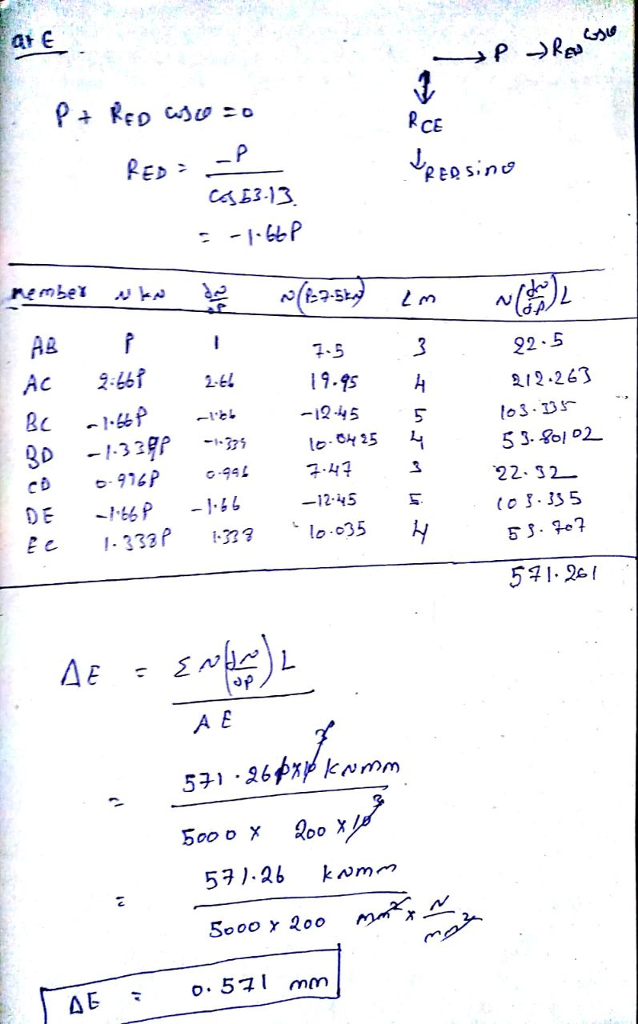#### Earn Coins

Coins can be redeemed for fabulous gifts.

Similar Homework Help Questions
• ### Question 1 (25%) For the truss shown on figure 1, determine the horizontal displacement of point...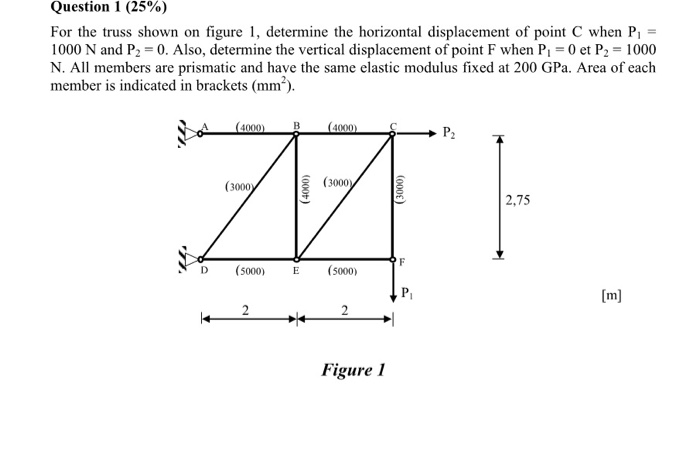Question 1 (25%) For the truss shown on figure 1, determine the horizontal displacement of point C when P 1000 N and P2 -0. Also, determine the vertical displacement of point F when P 0 et P2 1000 N. All members are prismatic and have the same elastic modulus fixed at 200 GPa. Area of each member is indicated in brackets (mm2). A (4000)B(400C (3000 (3000 2,75 D(5000 E(5000) Pi Figure 1

• ### Please show all work 4 kN 2. Use Castigliano's theorem to find the vertical displacement of...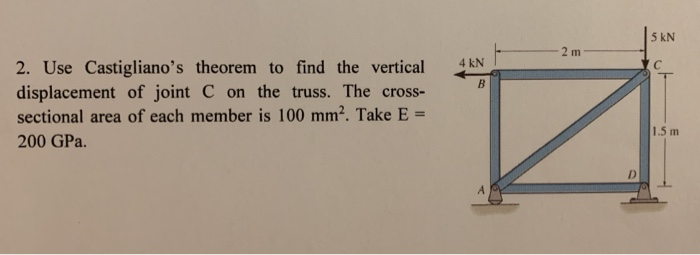Please show all work 4 kN 2. Use Castigliano's theorem to find the vertical displacement of joint C on the truss. The cross- sectional area of each member is 100 mm2. Take E = 200 GPa. 1.5 m

• ### Determine the vertical and horizontal displacement at F. Mernber Young's Modulus, E - 200 GPa Member...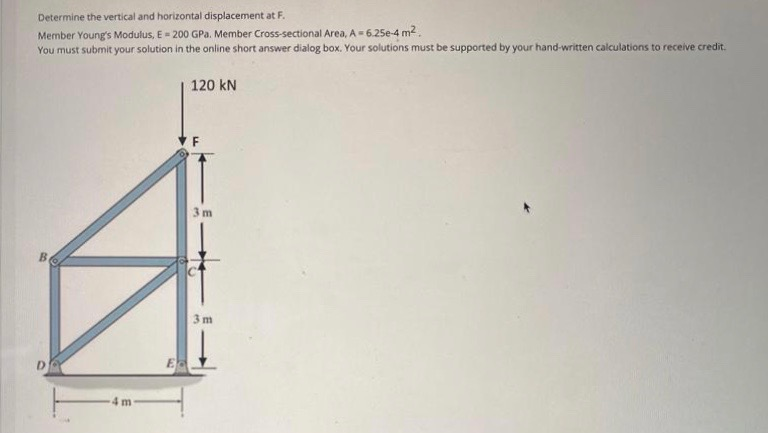Determine the vertical and horizontal displacement at F. Mernber Young's Modulus, E - 200 GPa Member Cross-sectional Area, A-6.25e-4 m2 You must submit your solution in the online short answer dialog box. Your solutions must be supported by your hand-written calculations to receive credit 120 KN F m B 3 m E. m

• ### 2. Determine the vertical displacement at joint B and horizontal displacement at joint D using Castigliano's...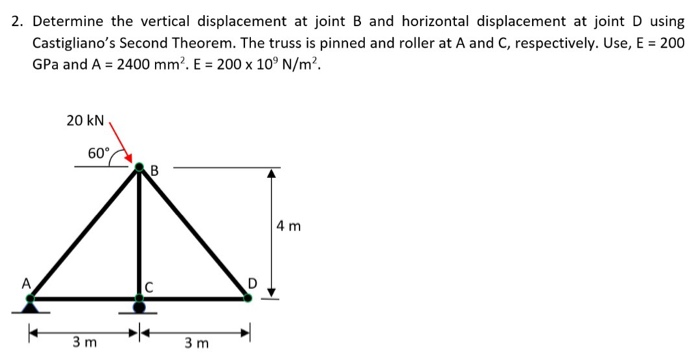2. Determine the vertical displacement at joint B and horizontal displacement at joint D using Castigliano's Second Theorem. The truss is pinned and roller at A and C, respectively. Use, E = 200 GPa and A = 2400 mm. E = 200 x 10°N/m². 20 KN 60 CAB 4 m 3m 3m

• ### Use the stiffness method to determine the horizontal and vertical components of the displacement at Joints...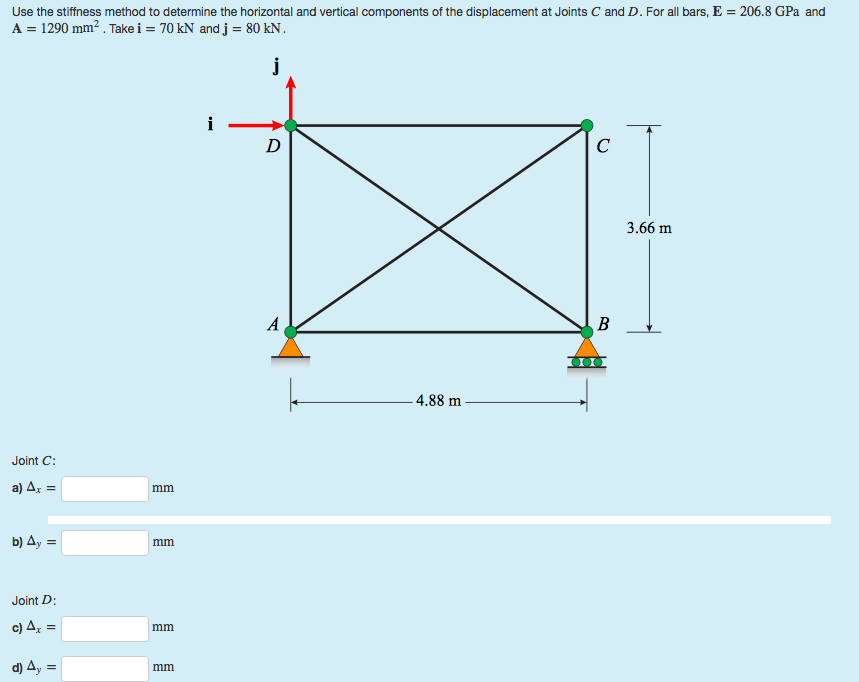Use the stiffness method to determine the horizontal and vertical components of the displacement at Joints C and D. For all bars, E = 206.8 GPa and A = 1290 mm². Take i = 70 kN and j = 80 kN. 3.66 m 4.88 m Joint C: a) Az = b) 4y = Joint D: c) 4, = d) 4, =

• ### Q3: Determine the vertical and horizontal displacement of joint A for the truss shown in Fig....Q3: Determine the vertical and horizontal displacement of joint A for the truss shown in Fig. (3). each bar is made of steel and has the cross-sectional area of 400mm Take E = 200 GPa Use the method of virtual work. E D 2 m |в -1.5 m -1.5 m 20 KN 40 KN Fig. (3)

• ### Q3: Determine the vertical and horizontal displacement of joint A for the truss shown in Fig....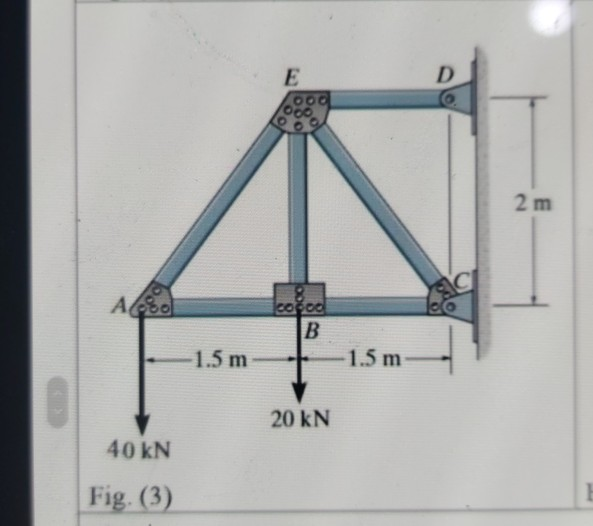Q3: Determine the vertical and horizontal displacement of joint A for the truss shown in Fig. (3), each bar is made of steel and has the cross-sectional area of 400mm?. Take E = 200 GPa. Use the method of virtual work. E D 00. 2 m СІ Ao cocoa B 1.5 m 1.5 m 20 KN 40 kN Fig. (3)

• ### 15 m B- Question 3: Each member of the truss shown is made of steel (E- 2 1 0 GPa ) and has a cro...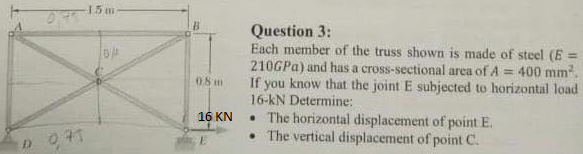15 m B- Question 3: Each member of the truss shown is made of steel (E- 2 1 0 GPa ) and has a cross-sectional area of A If you know that the joint E subjected to horizontal load 16-kN Determine: .The horizontal displacement of point E . The vertical displacement of point C. 400 mm2 0.8m 16 KN 15 m B- Question 3: Each member of the truss shown is made of steel (E- 2 1 0 GPa )...

• ### i need the answer quickly fast as you can Q3: Determine the vertical and horizontal displacement...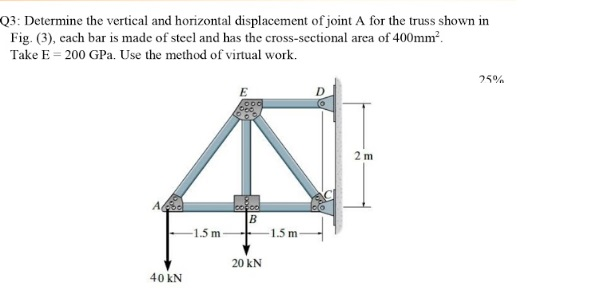i need the answer quickly fast as you can Q3: Determine the vertical and horizontal displacement of joint A for the truss shown in Fig. (3), cach bar is made of steel and has the cross-sectional area of 400mm? Take E = 200 GPa. Use the method of virtual work. 25% E 2 m А. cool B 1.5 m -1.5 m 20 KN 40 KN

• ### 4. Determine the vertical displacement of point C using Castigliano's theorem. Include axial displacements. Assume E,...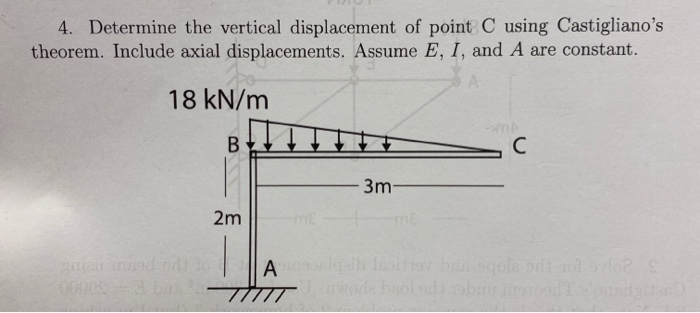4. Determine the vertical displacement of point C using Castigliano's theorem. Include axial displacements. Assume E, I, and A are constant. 18 kN/m 3m- 2m Alle